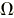# SI units

SI is abbreviation for Système International d’Unités (in French), in English it means International system of Units. It is the modern form of metric units and it is the most widely used system of measurement, accepted by most countries. It contains seven base units.

## SI base units

Unit name
Unit symbol
Quantity name
Dimension symbol
metre
m
length
l (lowercase L)
kilogram
kg
mass
m
second
s
time
t
ampere
A
electric current
I (uppercase i)
kelvin
K
thermodynamic temperature
T
mole
mol
amount of substance
n
candela
cd
luminous intensity
IV

## Named units derived from SI base units

Other quantities, called derived quantities, are defined in terms of the seven base units over different equations, like area (m×m=m2), speed (m/s) etc. Some derived units got special name, like Newton (N=kg×m/s2), Pascal (Pa = N/m2), etc.

Derived quantity Name Symbol Expression in terms of other SI units Expression in terms of SI base units
solid angle steradian sr   - m2·m-2 = 1
frequency hertz Hz   - s-1
force newton N   - m·kg·s-2
pressure, stress pascal Pa N/m2 m-1·kg·s-2
energy, work, quantity of heat   joule J N·m m2·kg·s-2
power, radiant flux watt W J/s m2·kg·s-3
electric charge, quantity of electricity coulomb C   - s·A
electric potential difference,
electromotive force
volt V W/A m2·kg·s-3·A-1
electric resistance ohmV/A m2·kg·s-3·A-2
electric conductance siemens S A/V m-2·kg-1·s3·A2
magnetic flux weber Wb V·s m2·kg·s-2·A-1
magnetic flux density tesla T Wb/m2 kg·s-2·A-1
inductance henry H Wb/A m2·kg·s-2·A-2
Celsius temperature degree Celsius °C   - K
luminous flux lumen lm cd·sr m2·m-2·cd = cd
illuminance lux lx lm/m2 m2·m-4·cd = m-2·cd
activity (of a radionuclide) becquerel Bq   - s-1
absorbed dose, specific energy (imparted), kerma gray Gy J/kg m2·s-2
dose equivalent sievert Sv J/kg m2·s-2
catalytic activity katal kat s-1·mol

Source: NIST.gov, SI base- and derived units physics.nist.gov/cuu/Units/units.html